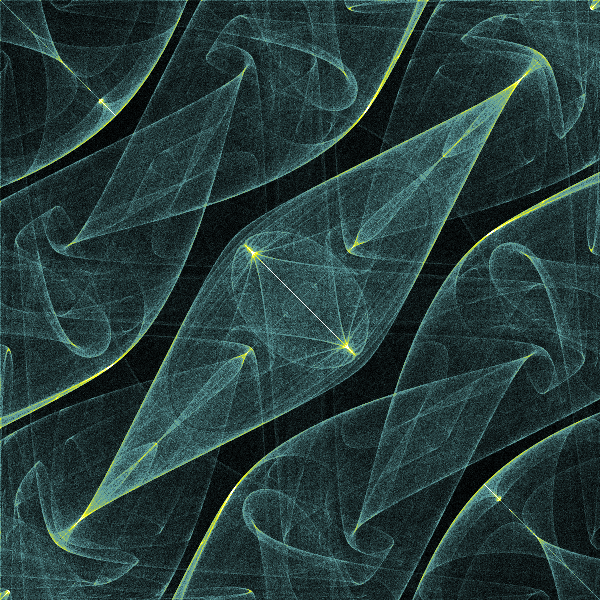# Popcorn fractal in generativepy

Martin McBride
2022-01-19

The Popcorn fractal is a strange attractor that is in some ways quite similar to the Hopalong fractal. Like the Hopalong fractal, is not a true attractor, because the pattern obtained depends on the initial values of x and y (in addition to the parameters H1, H2). However, the Popcorn fractal is not rendered in quite the same way as other strange attractors, as we will explain below.

It is worth reading the coloured Tinkerbell fractal article before tackling this fractal, to understand how to colour fractals.

## Popcorn equations

The fractal equations for Popcorn are:

xnext = x - H1*math.sin(y + math.tan(3*y))
ynext = y - H2*math.sin(x + math.tan(3*x))


where H1 and H2 are constants. The default value are 0.5 for both, but they can be varied to create different variants of the fractal.

## Initial values of x and y

With most strange attractors, we start with a particular x and y value, then run the algorithm lots of times, each time feeding the previous values back into the equations to generate the next set of points. We use an array to count the number of times the algorithm lands on each pixel. In the end we convert the count for each pixel into a colour.

For popcorn, we run the process above many times, using a different set of initial values each time. We count the number of times the algorithm lands on each pixel in total, across all the different initial values. This array of the total number of counts is then converted to a colour.

In our case, we run the algorithm once for every pixel in the output image, using the (x, y) position of the pixel as the starting value. For a 600 square image that means we run the attractor 360,000 times, each with a different start value. For each run, the attractor equations are executed 1000 times.

## The code

Here is the full code for the popcorn fractal:

from generativepy.bitmap import Scaler
from generativepy.nparray import make_nparray_data, save_nparray, load_nparray, make_npcolormap, apply_npcolormap, save_nparray_image
from generativepy.color import Color
from generativepy.analytics import print_stats, print_histogram
from generativepy.utils import temp_file
import math
import numpy as np

MAX_COUNT = 1000
H1 = 0.5
H2 = 0.5
WIDTH = 600
USERWIDTH = 2

def calc(x, y):
xn = x - H1*math.sin(y + math.tan(3*y))
yn = y - H2*math.sin(x + math.tan(3*x))
return xn, yn

def paint(image, pixel_width, pixel_height, frame_no, frame_count):
scaler = Scaler(pixel_width, pixel_height, width=USERWIDTH, startx=-USERWIDTH/2, starty=-USERWIDTH/2)

image[...] = 0

for i in range(0, WIDTH, 1):
for j in range(0, WIDTH, 1):
x, y = scaler.device_to_user(i, j)
for _ in range(MAX_COUNT):
x, y = calc(x, y)
px, py = scaler.user_to_device(x, y)
if 0 <= px < WIDTH and 0 <= py < WIDTH:
image[py, px] += 1

def colorise(counts):
counts = np.reshape(counts, (counts.shape, counts.shape))

colormap = make_npcolormap(int(np.max(counts))+1,
[50, 100, 100, 102400])

outarray = np.zeros((counts.shape, counts.shape, 3), dtype=np.uint8)
apply_npcolormap(outarray, counts, colormap)
return outarray

filename = temp_file('popcorn.dat')

data = make_nparray_data(paint, WIDTH, WIDTH, channels=1)
save_nparray(filename, data)

print_stats(data)
print_histogram(data)

frame = colorise(data)

save_nparray_image('popcorn.png', frame)


This code is available on github in blog/fractals/popcorn.py.

Here is the image it createsHere is the main loop:

    for i in range(0, WIDTH, 1):
for j in range(0, WIDTH, 1):
x, y = scaler.device_to_user(i, j)
for _ in range(MAX_COUNT):
x, y = calc(x, y)
px, py = scaler.user_to_device(x, y)
if 0 <= px < WIDTH and 0 <= py < WIDTH:
image[py, px] += 1


i and j loop over every pixel in the image. The scaler converts (i, j) in pixel space to (x, y) in image space to use as initial values. The inner loop executes each run of the fractal. Notice that MAX_COUNT is set to 1,000, compared to 10,000,000 that we used for Hopalong. This is necessary because we are executing the inner loop hundreds of thousands of times, and it would take a very long time to execute each loop 10 million times. Fortunately, because there are multiple runs, it isn't necessary to execute so many loops for each run.

## Variants

We can create variants of the Popcorn by changing the parameters H1, H2. We also change the scaling (via USERWIDTH) to view a different area of the space. Here are the new parameters:

MAX_COUNT = 1000
H1 = 0.4
H2 = 0.7
WIDTH = 600
USERWIDTH = 4


Here is the result:Obviously we have also changed the colour scheme. You can experiment with different values. This code is available on github in blog/fractals/popcorn2.py.